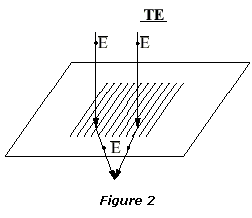# Influence of Polarization on Fringe Visibility

## Influence of illumination polarization on interference pattern fringe visibility

IBSEN Phase masks are optimized for the illumination polarization specified by the customer. The polarization does have an impact on the grating writing process, which is the subject of this Application Note. A Phase mask optimized for one polarization will perform differently if illuminated with a different polarization.

## TE and TM polarization modes defined, and requirements for interference

This section shortly describes the difference between TE and TM polarized light.

Figure 1 illustrates TE and TM polarization respectively of the light incident upon a Phase mask. The figure shows a +/-1 order Phase mask and its grating vector, which is perpendicular to the grating plane, and the incident light, which here is at normal incidence (Θ1=0) . The plane of incidence is the plane defined by the grating vector and the propagation vector of the incident light.

The light’s electromagnetic properties are defined by the orientation of its electric and magnetic fields. TE (Transverse Electric) polarized light is characterized by its electric field being perpendicular to the plane of incidence. For TE light, the magnetic field – always perpendicular to the electric field in isotropic materials – is parallel to the plane of incidence.

For TM (Transverse Magnetic) light, it is the magnetic field that is perpendicular to the plane of incidence, and hence the electric field is parallel to the plane of incidence.

The importance of the polarization characteristics is that only parallel oriented polarization modes interfere, and it is the interference between the +1 and -1 orders of the Phase mask that generates the grating in the fiber/waveguide.

## Fringe visibility using TE polarized light

As seen in figure 2, the polarization vectors of the 2 diffracted orders are parallel in the TE case. Hence the fringe visibility will be 100% (assuming full coherence of the light source).## Fringe visibility using TM polarized light

As seen in figure 3, for TM polarized light, the polarization vectors of the 2 diffracted orders are not parallel as in the TE case. Instead, there is an angle between them. This results in a fringe visibility that is less than 100%, as the degree of coherence now is less than 100%.The degree of coherence of the TM light is given by the interference angle between the two diffracted +/-1 orders. This angle is found from the grating equation:

m·λ = Λ (sinΘi + sinΘm)

where λ is the illumination wavelength, Λ is the center pitch of the Phase mask, Θi is the incidence angle and Θm is the angle of diffraction for each order m that satisfies the grating equation. At normal incidence (Θi=0), the diffracted angle will be:

Θm = sin-1(m·λ/Λ)

and the interference angle between the two orders is 2·Θm. When the TM light is divided into the interfering and non-interfering components (TM parallel and TM perpendicular), it is seen from cos and sin relations that the interfering light is cos2(2·Θm) and that the non-interfering light is sin2(2·Θm).

The fringe visibility of TM polarized light is therefore cos2(2·Θm).

## Fringe visibility using unpolarized light

The fringe visibility of unpolarized illumination can be evaluated as an average of the TE contribution and the TM contribution. The example below illustrates this evaluation, which is a typical example when writing FBGs for 1550 nm applications using an excimer laser at 248 nm:

### Example:Fringe visibility using unpolarized light

Λ = 1070 nm

λ = 248 nm

Phase mask principle = +/-1 order

Portion of TE light: 50% Portion of TM light: 50%

Fringe visibility for TE light: 100%

Interference angle = 2·sin-1(1*248/1070) = 27 degrees

Fringe visibility for TM light = cos2(27 degrees) = 79%

Total fringe visibility = (100% + 79%) / 2 = 90%

## Conclusion

As seen in the above example, the evaluation leads us to conclude that when a Phase mask is used with unpolarized illumination, the fringe visibility will be affected negatively. Whether the evaluated fringe visibility is sufficient, must be decided depending on the specific application.